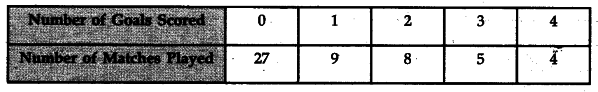# Calculate Coefficient of Variation from the following data :

Calculate Coefficient of Variation from the following data :